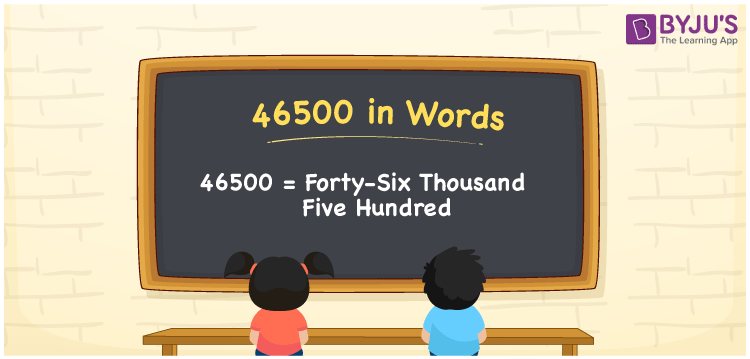# 46500 in Words

We can write 46500 in words as Forty-six thousand five hundred. If you charged Rs. 46500 to book a flight ticket, then you can say, “I booked a flight ticket for Forty-six thousand five hundred rupees”. We can convert the number 46500 into words with the help of a place value chart. Let’s learn how to convert the cardinal number 46500 in words, along with some facts about 46500, here in this article.

 46500 in words Forty-six thousand five hundred Forty-six thousand five hundred in Numbers 46500

## 46500 in English words

Generally, we express numbers in words using the English alphabet. So, we can spell 46500 in English as “Forty-six thousand five hundred”.## How to Write 46500 in Words?

To convert the number 46500 into words, we need to create a five-column place value chart since it has five digits. This can be done as given below.

 Ten thousand Thousands Hundreds Tens Ones 4 6 5 0 0

Here, ones = 0, tens = 0, hundreds = 5, thousands = 6, ten thousands = 4

Let us expand the above digits.

Thus, 4 × Ten thousand + 6 × Thousand + 5 × Hundred + 0 × Ten + 0 × One

= 4 × 10000 + 6 × 1000 + 5 × 100 + 0 × 10 + 0 × 1

= 40000 + 6000 + 500

= Forty thousand + Six thousand + Five hundred

= Forty-six thousand five hundred

Therefore, 46500 in words = Forty-six thousand five hundred.

### Facts About the Number 46500

As we know, 46500 is a natural number that is the successor of 46499 and the predecessor of 46501.

46500 in words – Forty-six thousand five hundred

Is 46500 an odd number? – No

Is 46500 an even number? – Yes

Is 46500 a perfect square number? – No

Is 46500 a perfect cube number? – No

Is 46500 a prime number? – No

Is 46500 a composite number? – Yes

## Frequently Asked Questions on 46500 in Words

Q1

### How do you write 46500 in English words?

The number 46500 can be written in words as Forty-six thousand five hundred.
Q2

### How to express Rs. 46500 in words on a cheque?

On a cheque, Rs. 46500 can be expressed in words as “Forty-six thousand five hundred rupees only”.
Q3

### Find 46500 – 30700. Express answer in words

46500 – 30700 = 15800 Therefore, the value of 46500 – 30700, i.e. 15800 in words is Fifteen thousand eight hundred.